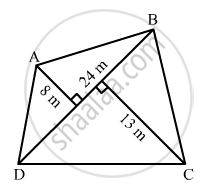# The Diagonal of a Quadrilateral Shaped Field is 24 M and the Perpendiculars Dropped on It from the Remaining Opposite Vertices Are 8 M and 13 M. Find the Area of the Field. - Mathematics

Sum

The diagonal of a quadrilateral shaped field is 24 m and the perpendiculars dropped on it from the remaining opposite vertices are 8 m and 13 m. Find the area of the field.

#### SolutionGiven:

Diagonal of a quadrilateral shaped field = 24 m
Perpendiculars dropped on it from the remaining opposite vertices are 8 m and 13 m.
Now, we know:
Area $= \frac{1}{2} \times d \times ( h_1 + h_2 )$
∴  Area of the field $= \frac{1}{2} \times 24 \times (8 + 13)$
$= 12 \times 21$
$= 252 m^2$
Concept: Area of a General Quadrilateral
Is there an error in this question or solution?

#### APPEARS IN

RD Sharma Class 8 Maths
Chapter 20 Mensuration - I (Area of a Trapezium and a Polygon)
Exercise 20.1 | Q 10 | Page 14